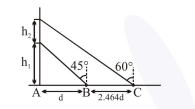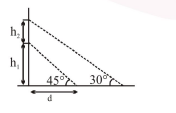# A balloon is moving up in air vertically above a point A on the ground.

Question:

A balloon is moving up in air vertically above a point $A$ on the ground. When it is at a height $\mathrm{h}_{1}$, a girl standing at a distance $\mathrm{d}$ (point $\mathrm{B}$ ) from A (see figure) sees it at an angle $45^{\circ}$ with respect to the vertical. When the balloon climbs up a further height $\mathrm{h}_{2}$, it is seen at an angle $60^{\circ}$ with respect to the vertical if the girl moves further by a distance $2.464 \mathrm{~d}$ (point C). Then the height $\mathrm{h}_{2}$ is (given $\tan 30^{\circ}=0.5774$ ) :1. $\mathrm{d}$

2. $0.732 \mathrm{~d}$

3. $1.464 \mathrm{~d}$

4. $0.464 \mathrm{~d}$

Correct Option: 1,

Solution:$\frac{\mathrm{h}_{1}}{\mathrm{~d}}=\tan 45^{\circ} \Rightarrow \mathrm{h}_{1}=\mathrm{d} \ldots$(1)

$\frac{\mathrm{h}_{1}+\mathrm{h}_{2}}{\mathrm{~d}+2.464 \mathrm{~d}}=\tan 30^{\circ}$

$\Rightarrow\left(\mathrm{h}_{1}+\mathrm{h}_{2}\right) \times \sqrt{3}=3.46 \mathrm{~d}$

$\left(\mathrm{h}_{1}+\mathrm{h}_{2}\right)=\frac{3.46 \mathrm{~d}}{\sqrt{3}}$

$\Rightarrow \mathrm{d}+\mathrm{h}_{2}=\frac{3.46 \mathrm{~d}}{\sqrt{3}}$

$h_{2}=d$